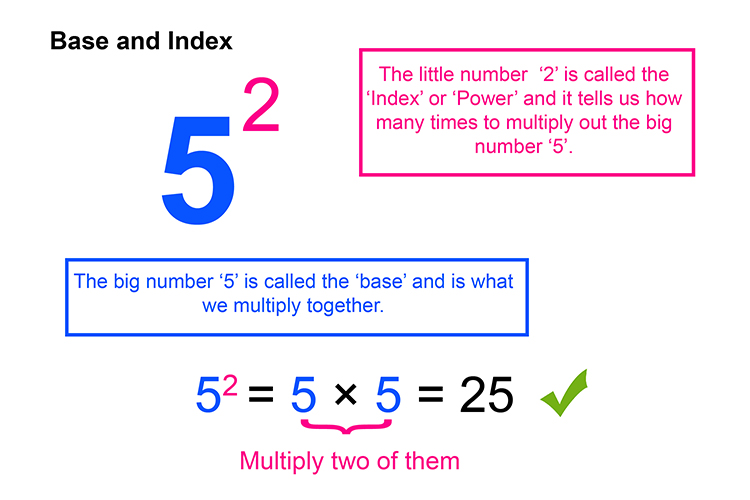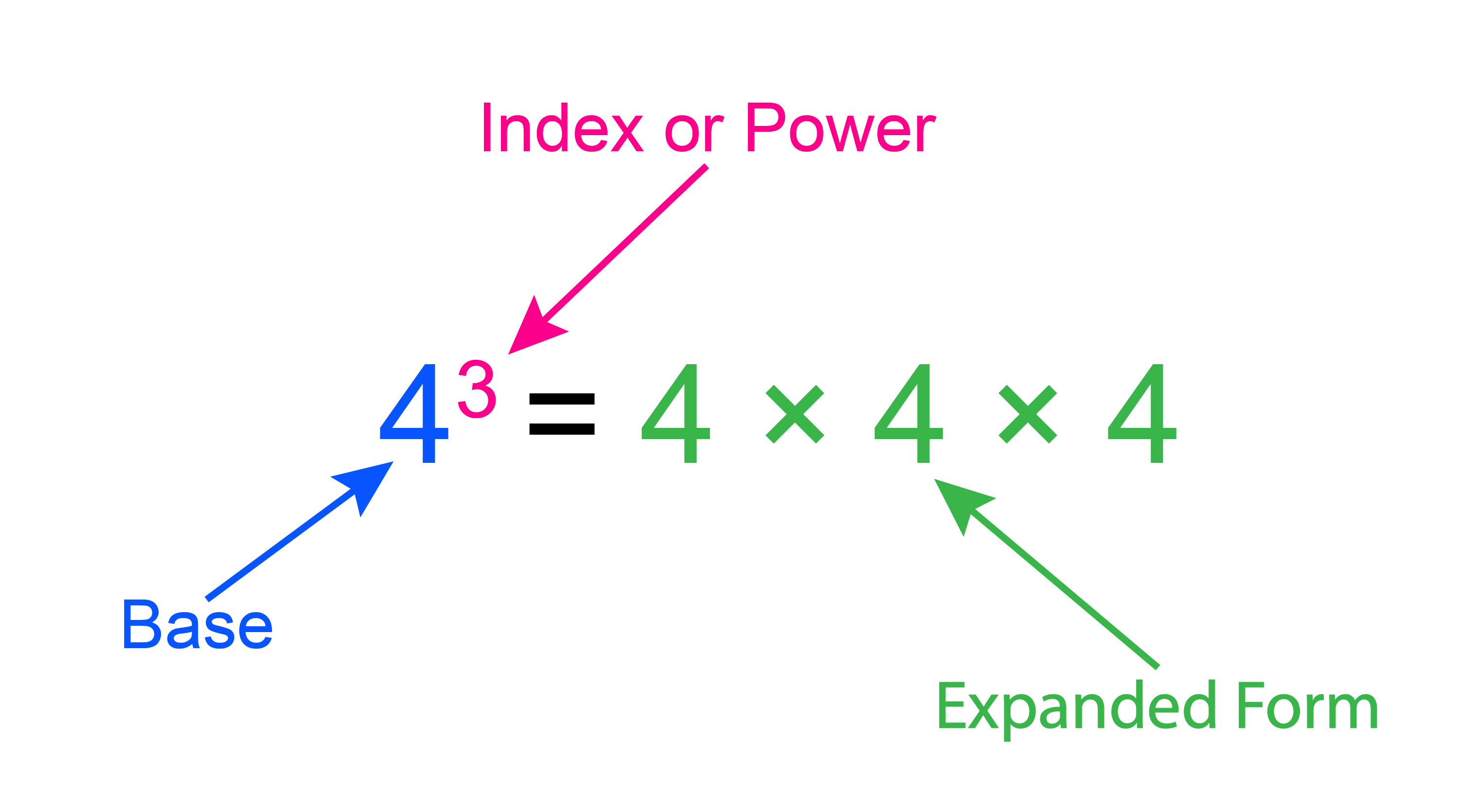# Powers and roots

## Indices – (or index) says how many times to use the number in a multiplication, called a POWER.In the seas, (indices) remember who has the POWER.

Example 1

5^4 = 5 to the power of 4

= 5\times5\times5\times 5 = 625

Example 2This is said to be 5 to the power of 2. When a number is to the power of 2 it is said to be squared.

Example 3This is said to be 4 to the power of 3 which is 4\times4\times4 = 64.

Example 4

9^(1/2)=sqrt9=3

Here 9 is to the power of a half, which is the same as the square root, which in this case is 3.

Example 5

x^2

This is x to the power of 2. Which is x squared, which is   x\ times\ x